# Aggregation of "percentages of total" and "percentages of sub-totals"

Fabian Koenig shared this question ago

Hi there!

I am looking to create a table as the following displaying revenue values and their percentage-distribution across all categories for:

a) each month

and

b) for the yearly total

If in any way possible, this should also work then for sub-totals:(i) In the upper table the percentage add up to 100% on the sub-total row. I would also be happy having each of the sub-total tables as separate sections.

(ii) In the lower table, on the other hand, the percentages add up to 100% in the grand total row.

You'll notice that also the yearly totals amount to 100% on the respective levels (i.e. sub-totals or grand totals) - it sums each category across all months of the year, and then calculates the %-distribution of each category in the yearly totals.

So far I have only been able to get a yearly total which will have e.g. 1200%, i.e. summing up the percentages of each month in the yearly total, which IMHO doesn't really make any sense (at least I haven't found a use case where this logic would render valuable insight).

I've also looked at Nathan's awesome plugin featured under https://community.yellowfinbi.com/topic/percentage-of-sub-total which will get me some way towards the upper table, however, the yearly totals are something I can't yet figure out how to accomplish.

How can I achieve this ?

Thank you & Best Regards

Fabian1

Hi Fabian,

It looks like Nathan is assisting you with this in the pre-existing linked Percentage of Sub-Total community Item (https://community.yellowfinbi.com/topic/percentage-of-sub-total), so I'm going to go ahead and close this question out.

Regards,

Mike1

Hi Mike,

no, this is a separate inquiry with a different target - could you please re-open the ticket.

The linked item (https://community.yellowfinbi.com/topic/percentage-of-sub-total) was evolving around a fatal error due to incompatible java versions.

This topic is around creating a report in specific manner with percentages totalling across the yearly totals as opposed to summing up the percentages of the individual months.

Thank you & Best Regards

Fabian1

Hi Fabian,

Thank you for clarifying. Generally, support handles requests related to defective behavior within the application. Report building and creation such as this falls more in the realm of consulting services. That said, I can provide a pointer or two, if I understand your query correctly.

If you're referring to a total of all the values in the last column, like so: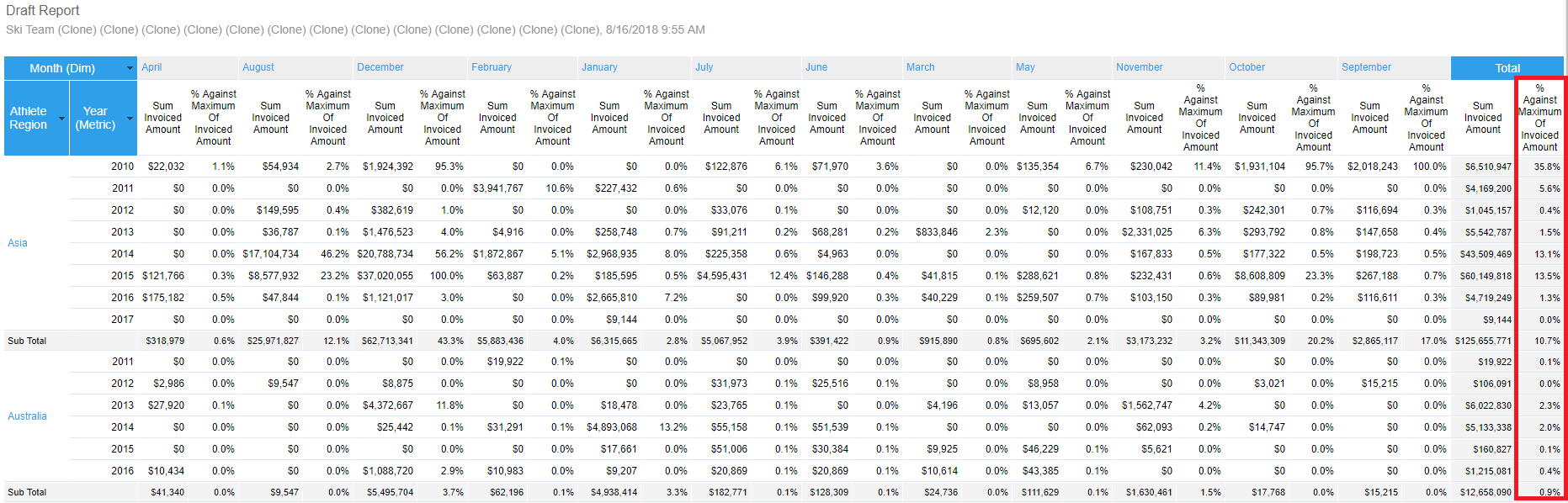Then you can just set the corresponding percentage Advanced Function Totals to Average, instead of Sum: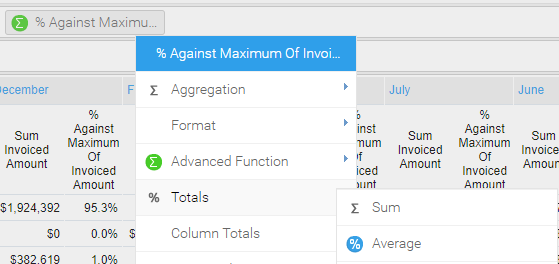If I set this field to Sum, then it adds up all the percentages instead, so if I had 12 values of 100% it would show as 1200% instead of 100%, which of course wouldn't make sense. It looks like your '2017 Total' column from what looks like Excel is averaging all the values, so I believe this is what you're looking for.

If not, please provide some screenshots from in YF itself of what you've come up with so far.

Regards,

Mike1

Hi Mike,

here are some screen shots of the odd behavior of Yellowfin based on the SkiTeam sample data:

1.) here you see the sums of invoiced amounts for a select few camps.

• the %-distribution in each year nicely adds up to 100% in the bottom line => perfect.
• however, the Total column brings bogus. Here the total adds up to 600% - which does not make _any_ sense. The %-distribution should be calculated as ["Total Invoiced" / "Total of Total Invoiced"], so for example in the first line: 168.768/1.332.680 = 12,66%. Instead, it amounts to 224% which does not make any sense at all.• By the way: I also noticed, although I have selected "Percentage of Total" as the advanced function, I do not get real percentage values but instead percentage*100 - I therefore have to format the column as "Numeric" with "suffix %" as opposed to formatting it as "Percentage".The summary in this example is set to the following:

• it correctly sums the columns up to 100%, which is desired outcome.2.) Now, following your suggestion, I have switched from total aggregation "sum" to "average":and the result brings complete bogus:as you will see:

• the totals in the columns are no longer adding up to 100% as soon as there are multiple camps with invoices for them.
• Also the percentages in the Total column don't make any sense.

To the statement that my my "'2017 Total' column from what looks like Excel is averaging all the values": no, it is not averaging, but it is calculating the percentage-distribution for each row in each period - and it is doing the same for the "total" period, which is the sum of all periods with regards to the revenue values, and then calculates what percentage each row-total has compared to the overall total.

While I fully appreciate that report building falls into the realm of consulting services, the fact that a "percentage of total" totals up to anything else than 100% just doesn't make any sense to me and imho appears to me as a buggy behavior.

Thank you very much for your support & Best Regards

Fabian1

Hi Fabian,

Thanks for your response. The behavior being shown here does make sense in terms of the underlying logic. I believe the confusion likely stems from the fact that when you're choosing Totals the same calculation is applied to both the Row and Column totals.

First screenshot: The row total is 224 because when using 'Totals > SUM' this value equals 224... Reading left to right on first row, 100 + 100 + 24 + 0's = 224. It's 100 as the Column total, because 100 + all 0's is 100. The %-distribution would not be 12.66, it'd be 75%, as 100 + 100 + 24 = 224 / 3 = 74.66...

Second screenshot: That is correct. This is because it is applying a function on top of numeric data. In order to properly make use of the percent formatter, you'd want your underlying data to already be percentage values, otherwise it will not apply functions to the data as expected. I'm probably not explaining this aspect too well, but the important thing to focus on and take note of here is whether the values of the math are actually correct.

Third screenshot: Like in the first screenshot, the SUM is 100 because that happens to be the actual SUM of the given column values.

Fourth & Fifth Screenshots: when choosing 'Totals > Average', it's just averaging non-0 values. For example, in the 2012 column, 76 + 24 = 100 / 2 = 50. In the 2014 column, 42 + 4 + 53 = 99 / 3 = 33 (values differ due to rounding using 0 decimal places).

Hopefully this clears this up a bit and also makes it more understandable in terms of why, given the logic involved, it's not as straight forward a solution to accomplish what you're after as one would probably think, which is completely understandable for the record, as it's not incredibly intuitive. It takes months of dealing with queries such as these to begin to think this way by default and begin to intuit it. Along with that comes an understanding that while this may be possible, it'd be quite difficult to actually achieve this as you can't apply to separate Total Aggregations to the same field in different places, or that actually, it may be the case that this would require a Percentage of Total ran against the Sum Total column itself, on a per row basis.

Ultimately though, I can't really spend more time with this as it's outside the scope of support and I've already been trying to figure this out for at least a couple of hours. Sorry I am unable to assist further with this, but hopefully this at least clears up some of the confusion on what's actually occurring regarding the functions and math here which will maybe spark some ideas to accomplish what you're after.

Regards,

Mike1

Hi Mike,

However, I cannot believe that Yellowfin is incapable of providing a break-down of numbers as "percentages of the total", which will work properly on the detail-level (in this example individual years) as well as the summary-level (in this example all years from 2010 till 2016).

And I can even less believe that no other user of the application is heavily demanding this.

This seems to be a clear flaw in the inderlying logic.

Thank you again for your support in this. However, it might be beneficial if we could ask Nathan to throw a quick eye on the matter. I believe we are somehow talk cross-purposes here and I'm positive he'd immediately be able to relate to the problem at hand.

You stated:

`First screenshot: [...] It's 100 as the Column total, because 100 + all 0's is 100. `
sorry, no, this is not correct. Look at year 2014:

• Here you have \$212.047 in Italy which equals to 42% of the 2014-total of \$501.068 (\$212.047 / \$501.068 = 42,32%).
• You also have \$21.402 in Sri Lanka which equals to 4% of the 2014-total (\$21.402 / \$501.068 = 4,27%).
• And you also have \$267.620 in Sweden which equals to 53% of the 2014-total (\$267.620 / \$501.068 = 53,41%).

In the 2014-total these 3 percentages add up to 100% = 42,32% + 4,27% + 53,41%

This makes perfect sense and it has nothing to do with the assumption that there would be only 1 value of 100% and all other values are 0%.

The calculation of the percentages within each year-column as well as the aggregation of the row-values in the column-total of each year is correct, exactly as expected and spot on to what I described above.

However, the calculation of the percentages across the years is simply wrong.

The values displayed in the TOTAL column are NOT "Percentages of Total". They are, instead, "Sum of annual percentages from annual totals".

Hence, the mathematical logic underlying the %-values displayed in the yearly columns is not the same as the mathematical logic underlying the %-values displayed in the TOTAL column.

The total column would have to be calculated as

`sum_across_years ( yearly_revenue_of_current_row ) /  sum_across_years ( yearly_revenue_total )`

`sum_across_years ( yearly_revenue_of_current_row / yearly_revenue_total )`

Sorry, it's just plain wrong.

Please note here that yearly_revenue_of_current_row in itself is an aggregation of numerous invoices which fall in to the categorization of that particular row and that particular year.

I cannot see that the results provided by the current mathematical logic would be beneficial in any use case whatsoever.

Thank you & Best Regards

Fabian1

Hi Fabian,

Thanks for your reply. I think the disconnect/confusion is on how and where Totals are being applied. Row Totals follow the aggregations applied to the given Metric(s).

I don't think Yellowfin is incapable of providing a break-down of numbers as percentages of the total, it's just that, as stated in an earlier reply, this may be possible via a freehand SQL query, calculated fields, custom functions, and/or inserting new columns into the db, but it's not going to be as straightforward as toggling a Totals option. The value in the final Total column is not itself an actual % of Total of Invoiced Amount. I also agree that there may not be a good use case for the Rows being totaled in this way in this example, but this is merely an example of a row total being applied to an Advanced Function. Because of the aforementioned, in terms of demand, it's quite difficult to how many users are doing this, as this is not the type of question support is intended to field, as the focus is on defective behavior, which this is not, which I'm hoping my forthcoming breakdown will clear up.

Here is me turning on Row Totals for Camp Demographic, using Totals > SUM. Invoiced Amount is also set to SUM: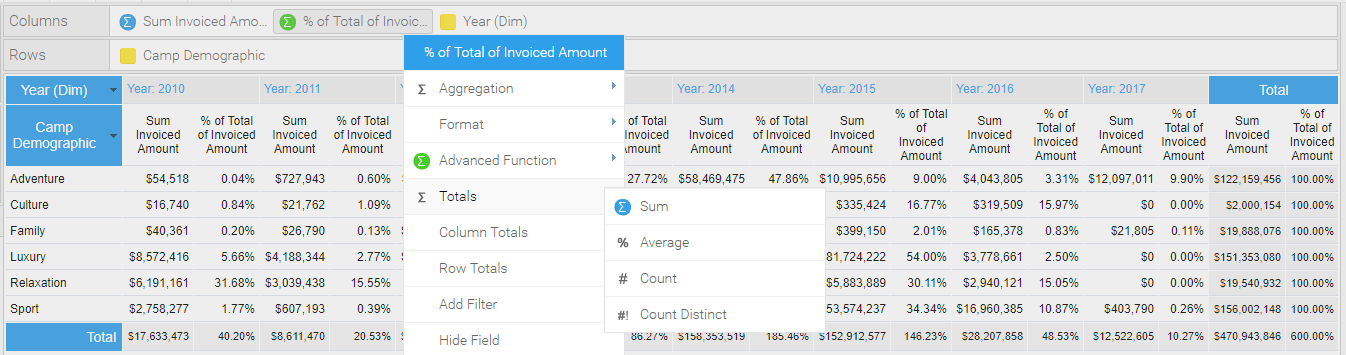The sum of the values from left to right, i.e., the values of the Row, where the totals are being applied to, total to 100%. The same aggregation applies to the Column Total on each Year as well, i.e., 2010's Column Total is 40.20%.

If I switch to Totals > Average for the '% Total of Invoiced Amount' field, it now outputs an average for the corresponding row in the Totals sections, while Invoiced Amount is still showing the SUM, as I have not changed that one: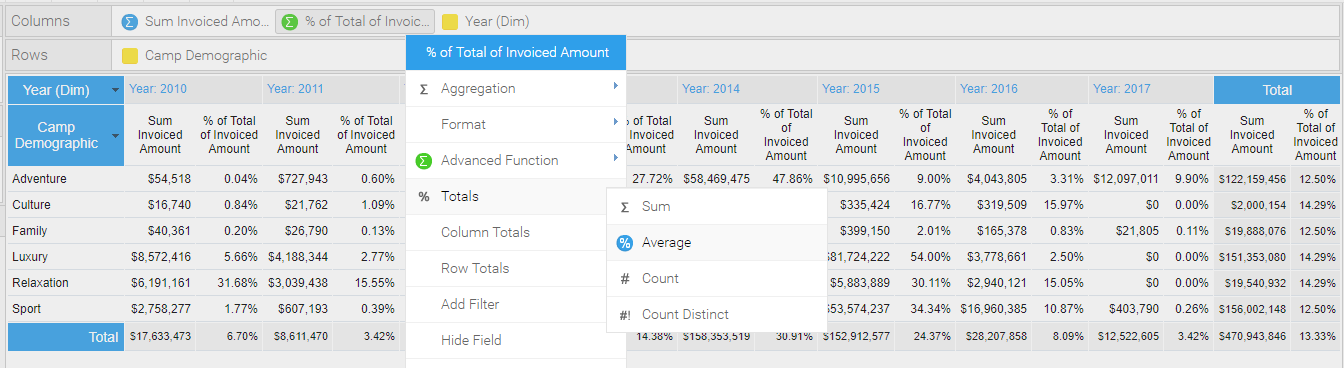You can now see Row Totals displayed, which, following the aggregations of the Metrics here, means it is summing up the values left to right. This is indeed the query being generated:

```sum_across_years ( yearly_revenue_of_current_row / yearly_revenue_total )
```
Which is to be expected. I conferred with Nathan on these points and he is in agreement this is expected behavior here.

Regarding this: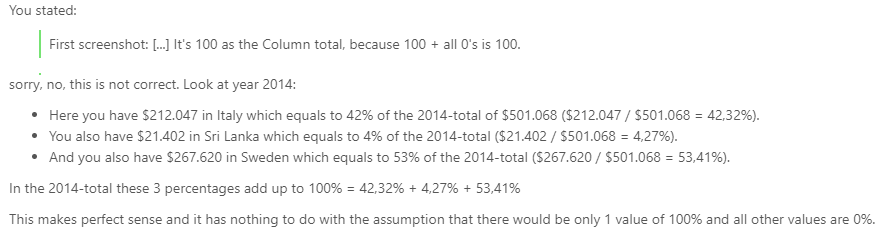I am referring to the sum of the values in the Column for 2010, which is 100 + 0 + 0 + 0 + 0 + 0 = 100, in your example. There's no assumption that all other values are 0.. aside from Austria the reamining values are all 0's in the data you used as an example. For 2014, this too equals 100: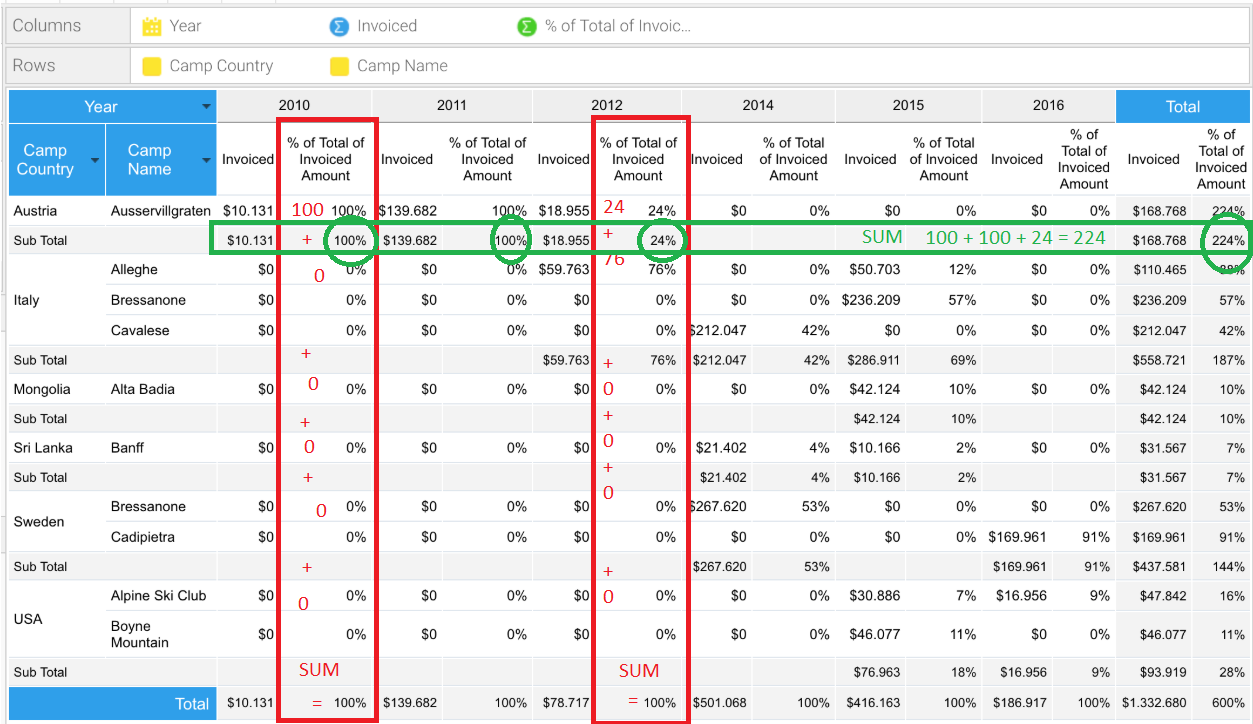"The values displayed in the TOTAL column are NOT "Percentages of Total". They are, instead, whichever aggregation you apply to your Metrics which will then be calculated when applying Row and Column Totals. All of this is working as expected.

To get to what you're after, you already gave a condensed, math logic version of what you're looking for as your Total column:

`sum_across_years ( yearly_revenue_of_current_row ) /  sum_across_years ( yearly_revenue_total )`
The trick now is to manipulate and plug-in your data to make this a SQL logic statement (via a Calculated Field most likely), so that it is output correctly within the application. The precise scripting of which is what I was referring to as being outside the scope of support, because as you can see, if you actually try and write a SQL query for even the 'sum_across_years' element, you can see it's not really going to wind up being 4 parts, and will contain more than 4 elements, getting increasingly complex the more each aspect interacts with one another. Each of the 4 parts you've described here are going to have to be broken down further and will thus likely end up being a pretty complex query. Perhaps if you have a dev on hand they may be able to assist in drafting this.

I think this all may have become more convoluted than it needed to be. We had one issue whereby the confusion was regarding Totaling, or more specifically, one of the Totals being labeled as '% of Total Of Invoiced Amount', although the values are just aggregations of the Row data; and two, that accomplishing what you're actually after here is going to require a complex query we are unable to assist with.

Considering these points I this clears this all up maybe, haha. Please don't hesitate to reach out for further clarity if need be, however.

Regards,

Mike1

Hi Mike,

I have taken the freedom to spend 5 minutes drafting a similar table in Tableau Public. As you will see, Tableau is capable of immediately providing exactly the details required for the desired "percentage of total", summing up correctly in all the aggregation columns (total per quarter, total per year, etc)In tableau you'd simply add a quick-table function "Percent of Total":and specify along which dimension you want to calculate the totals - in this case we want to total across the states, so that each month, each quarter and each year column total to 100%.As you can see, in Tableau there are no Total-Columns with a "Percentage of Total" above 100% - each column is correctly calculated.

This is what I expect from a BI solution - and I just can't believe that in Yellowfin you'd have to perform elaborate SQL tricks in order to achieve this very basic query.

I could provide the same samples from SAP BI and probably any other reasonably powerful BI - and it should be a task of minutes if not seconds to achieve this.

And, despite I'm probably repeating myself, the "percentages of total" values which Yellowfin produces in the TOTAL columns, simply do not make any sense. They also don't make any sense in the example you provided above with regards to Camp Demographics. The values completely lose their meaning as soon as the individual percentages are simply aggregated by summing or averaging them.

And just to be sure we're not in misunderstanding here: I am not arguing that the result of adding up the individual percentages for example in the last image you posted would not amount to 224% in the Austria Sub-Total row. Clearly, it would - what I am saying, however, is: summing up the individual percentages renders a number which has no meaning anymore, because it is losing its reference. From a business perspective, such an aggregation is of no value. From a business perspective you would always have to calculate the percentage within the total column by diving the row-total through the overall total, and there is no other way of arriving at the correct result that applying this mathematical logic. You will never succeed with averaging or summing individual percentage values.

I still have the feeling we are somehow talking cross-purpose, because this is such a basic query that I simply cannot believe a BI solution like Yellowfin is incapable of providing a direct solution for this.

Thank you once again & Best Regards

Fabian1

Hi Fabian,

Thanks for your response. I see what you're saying. I suppose it is pretty surprising that this isn't a heavily requested feature when considering this is a Quick Table Calculation option in Tableau.

I've gone ahead and submitted an enhancement request to add a "Percentage of Total Row Total Option", because as we've determined here, if you set the Aggregation to Average, then the Column Totals no longer make sense in terms of using a Percent of Total Advanced Function, and if you use SUM, it adds up the percentages, which also isn't useful.

Ultimately, I think it the case that Percent of Total is relatively unique when considering the other Advanced Function options, and that it may need to have its own set of options to Total in a desirable way.

Unfortunately, there's no way I can even think of of approximating the look of your sample reports since the Totals column is simply not going to give you what you're looking for here. It seems necessary this would have to be added as a feature.

I've created a public Idea in our Community to track this: https://community.yellowfinbi.com/topic/add-a-percent-of-total-row-totals-option.

Please reference that Community item for any potential future updates regarding this and please let me know if you have any further questions.

Regards,

Mike1

Hi Mike,

thank you very much. I'm glad the confusions could be settled and we're in agreement that there is something missing at the moment.

I'm very much looking forward to this getting implemented, hopefully not too far down the line.

Can you give any estimate as to when we could expect this feature? Or will it depend predominantly on how many votes the idea gets?

Thank you & Best Regards

Fabian1

Hi Fabian,

You're welcome. I'm glad too! Haha. I couldn't give a good or valid estimate due to the varying nature of developing certain features.

However, what I can say for certain is that 8.0 is coming in a month and aside from show-stopper critical defects, all dev focus is currently on that. Beyond this, it's also been announced internally here that the next build of 7.4 will not to be released until December. As such, December would be the best case for this to be implemented, should it be chosen for development.

Anyways, I'm going to go ahead and mark this as Answered as all future updates regarding this will be posted in that community Idea. I keep on top of updates in our internal task that are linked to my name, so I'll let you know of any status updates promptly.

Please don't hesitate to reach out with any other questions or concerns.

Regards,

Mike1

GREAT thread here. I have used Tableau since 2012, and I LOVE Tableau. However, I know that not everyone can afford such a solution for an enterprise-level need. Currently we are using YellowFin BI with EHR solution. This thread speaks directly to an issue I had last week with respect to productivity % reports I've been building in YellowFin. Thank you for all the time/effort in documenting an updating this post = WONDERFUL!

- Mo1

Hi Mo,

Thanks for your feedback on this.

On a related side note, there are no further updates on this at this time.

Regards,

Mike